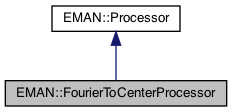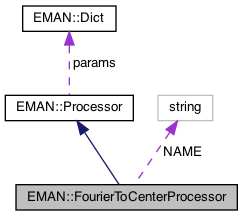EMAN2
EMAN::FourierToCenterProcessor Class Reference

Translates the origin in Fourier space from the corner to the center in y and z Handles 2D and 3D, and handles all combinations of even and oddness Typically you call this function after Fourier transforming a real space image. More...

`#include <processor.h>`

Inheritance diagram for EMAN::FourierToCenterProcessor:[legend]
Collaboration diagram for EMAN::FourierToCenterProcessor:[legend]

## Public Member Functions

virtual void process_inplace (EMData *image)
Fourier origin shift the image in the forward direction. More...

virtual string get_name () const
Get the processor's name. More...

virtual string get_desc () const
Get the descrition of this specific processor. More...Public Member Functions inherited from EMAN::Processor
virtual ~Processor ()

virtual EMDataprocess (const EMData *const image)
To proccess an image out-of-place. More...

virtual void process_list_inplace (vector< EMData * > &images)
To process multiple images using the same algorithm. More...

virtual Dict get_params () const
Get the processor parameters in a key/value dictionary. More...

virtual void set_params (const Dict &new_params)
Set the processor parameters using a key/value dictionary. More...

virtual TypeDict get_param_types () const
Get processor parameter information in a dictionary. More...

## Static Public Member Functions

static ProcessorNEW ()Static Public Member Functions inherited from EMAN::Processor
static string get_group_desc ()
Get the description of this group of processors. More...

static void EMFourierFilterInPlace (EMData *fimage, Dict params)
Compute a Fourier-filter processed image in place. More...

static EMDataEMFourierFilter (EMData *fimage, Dict params)
Compute a Fourier-processor processed image without altering the original image. More...

## Static Public Attributes

static const string NAME = "xform.fourierorigin.tocenter"Public Types inherited from EMAN::Processor
enum  fourier_filter_types {
TOP_HAT_LOW_PASS , TOP_HAT_HIGH_PASS , TOP_HAT_BAND_PASS , TOP_HOMOMORPHIC ,
GAUSS_LOW_PASS , GAUSS_HIGH_PASS , GAUSS_BAND_PASS , GAUSS_INVERSE ,
GAUSS_HOMOMORPHIC , BUTTERWORTH_LOW_PASS , BUTTERWORTH_HIGH_PASS , BUTTERWORTH_HOMOMORPHIC ,
KAISER_I0 , KAISER_SINH , KAISER_I0_INVERSE , KAISER_SINH_INVERSE ,
SHIFT , TANH_LOW_PASS , TANH_HIGH_PASS , TANH_HOMOMORPHIC ,
}
Fourier filter Processor type enum. More...Protected Attributes inherited from EMAN::Processor
Dict params

## Detailed Description

Translates the origin in Fourier space from the corner to the center in y and z Handles 2D and 3D, and handles all combinations of even and oddness Typically you call this function after Fourier transforming a real space image.

After this you operate on the Fourier image in convenient format, then you call FourierToCornerProcessor (above) and then inverse FT to get to the original image

Date
October 2007

Definition at line 6643 of file processor.h.

## ◆ get_desc()

 virtual string EMAN::FourierToCenterProcessor::get_desc ( ) const
inlinevirtual

Get the descrition of this specific processor.

This function must be overwritten by a subclass.

Returns
The description of this processor.

Implements EMAN::Processor.

Definition at line 6663 of file processor.h.

6664 {
6665 return "Translates the origin in Fourier space from the corner to the center in y and z - works in 2D and 3D";
6666 }

## ◆ get_name()

 virtual string EMAN::FourierToCenterProcessor::get_name ( ) const
inlinevirtual

Get the processor's name.

Each processor is identified by a unique name.

Returns
The processor's name.

Implements EMAN::Processor.

Definition at line 6653 of file processor.h.

6654 {
6655 return NAME;
6656 }
static const string NAME
Definition: processor.h:6668

References NAME.

## ◆ NEW()

 static Processor * EMAN::FourierToCenterProcessor::NEW ( )
inlinestatic

Definition at line 6658 of file processor.h.

6659 {
6660 return new FourierToCenterProcessor();
6661 }
Translates the origin in Fourier space from the corner to the center in y and z Handles 2D and 3D,...
Definition: processor.h:6644

## ◆ process_inplace()

 void FourierToCenterProcessor::process_inplace ( EMData * image )
virtual

Fourier origin shift the image in the forward direction.

Parameters
 image the image to operate on
Exceptions
 ImageFormatException if the image is not complex

Implements EMAN::Processor.

Definition at line 6013 of file processor.cpp.

6014{
6015// if ( !image->is_complex() ) throw ImageFormatException("Can not Fourier origin shift an image that is not complex");
6016
6017 int nx=image->get_xsize();
6018 int ny=image->get_ysize();
6019 int nz=image->get_zsize();
6020
6021 int nxy = nx*ny;
6022
6023 if ( ny == 1 && nz == 1 ){
6024 cout << "Warning- attempted Fourier origin shift a 1D image - no action taken" << endl;
6025 return;
6026 }
6027
6028 int yodd = (ny%2==1);
6029 int zodd = (nz%2==1);
6030
6031 float* rdata = image->get_data();
6032
6033 float tmp;
6034 float* p1;
6035 float* p2;
6036
6037 // This will tackle the 'normalization' images which come out of the Fourier reconstructor.
6038 // ie- real-space 1/2 FFt images centered on the corner
6039 if ( !image->is_complex() ) {
6040 if (nz!=1 && !yodd && !zodd) {
6041 for (int x=0; x<nx; x++) {
6042 for (int y=0; y<ny; y++) {
6043 for (int z=0; z<nz/2; z++) {
6044 int y2=(y+ny/2)%ny;
6045 int z2=(z+nz/2)%nz; // %nz should be redundant here
6046 size_t i=x+y*nx+(size_t)z*nxy;
6047 size_t i2=x+y2*nx+(size_t)z2*nxy;
6048 float swp=rdata[i];
6049 rdata[i]=rdata[i2];
6050 rdata[i2]=swp;
6051 }
6052 }
6053 }
6054
6055 return;
6056 }
6057 else throw ImageFormatException("Can not Fourier origin shift an image that is not complex unless it is even in ny,nz and nx=ny/2+1");
6058 }
6059
6060 if (yodd){
6061 // In 3D this is swapping the bottom slice (with respect to the y direction) and the middle slice,
6062 // shifting all slices below the middle slice down one. In 2D it is equivalent, but in terms of rows.
6063 float prev;
6064 size_t idx;
6065 for( int s = 0; s < nz; s++ ) {
6066 for( int c =0; c < nx; c += 2 ) {
6067 idx = (size_t)s*nxy+c;
6068 prev = rdata[idx];
6069 prev = rdata[idx+1];
6070 for( int r = ny/2; r >= 0; --r ) {
6071 idx = (size_t)s*nxy+r*nx+c;
6072 float* p1 = &rdata[idx];
6073 tmp = p1;
6074 tmp = p1;
6075
6076 p1 = prev;
6077 p1 = prev;
6078
6079 prev = tmp;
6080 prev = tmp;
6081 }
6082 }
6083 }
6084 }
6085
6086 // 3D - Shift slices correctly in the y direction, 2D - shift rows
6087 size_t idx1, idx2;
6088 for( int s = 0; s < nz; ++s ) {
6089 for( int r = 0; r < ny/2; ++r ) {
6090 for( int c =0; c < nx; c += 2 ) {
6091 idx1 = (size_t)s*nxy+r*nx+c;
6092 idx2 = (size_t)s*nxy+(r+ny/2+yodd)*nx+c;
6093 p1 = &rdata[idx1];
6094 p2 = &rdata[idx2];
6095
6096 tmp = p1;
6097 tmp = p1;
6098
6099 p1 = p2;
6100 p1 = p2;
6101
6102 p2 = tmp;
6103 p2 = tmp;
6104 }
6105 }
6106 }
6107
6108 if ( nz != 1 ) {
6109 if (zodd){
6110 // Swap the front slice (with respect to the z direction) and the middle slice
6111 // shifting all slices behind the middles slice towards the front slice 1 voxel.
6112 float prev;
6113 size_t idx;
6114 for( int r = 0; r < ny; ++r ) {
6115 for( int c =0; c < nx; c += 2 ) {
6116 prev = rdata[r*nx+c];
6117 prev = rdata[r*nx+c+1];
6118 for( int s = nz/2; s >= 0; --s ) {
6119 idx = (size_t)s*nxy+r*nx+c;
6120 float* p1 = &rdata[idx];
6121 tmp = p1;
6122 tmp = p1;
6123
6124 p1 = prev;
6125 p1 = prev;
6126
6127 prev = tmp;
6128 prev = tmp;
6129 }
6130 }
6131 }
6132 }
6133
6134 // Shift slices correctly in the y direction
6135 size_t idx1, idx2;
6136 for( int s = 0; s < nz/2; ++s ) {
6137 for( int r = 0; r < ny; ++r ) {
6138 for( int c =0; c < nx; c += 2 ) {
6139 idx1 = (size_t)s*nxy+r*nx+c;
6140 idx2 = (size_t)(s+nz/2+zodd)*nxy+r*nx+c;
6141 p1 = &rdata[idx1];
6142 p2 = &rdata[idx2];
6143
6144 tmp = p1;
6145 tmp = p1;
6146
6147 p1 = p2;
6148 p1 = p2;
6149
6150 p2 = tmp;
6151 p2 = tmp;
6152 }
6153 }
6154 }
6155 }
6156 image->set_shuffled(true);
6157}
#define rdata(i)
Definition: analyzer.cpp:592
#define ImageFormatException(desc)
Definition: exception.h:147
#define y(i, j)
Definition: projector.cpp:1516
#define x(i)
Definition: projector.cpp:1517

References ImageFormatException, rdata, x, and y.

## ◆ NAME

 const string FourierToCenterProcessor::NAME = "xform.fourierorigin.tocenter"
static

Definition at line 6668 of file processor.h.

Referenced by get_name().

The documentation for this class was generated from the following files: